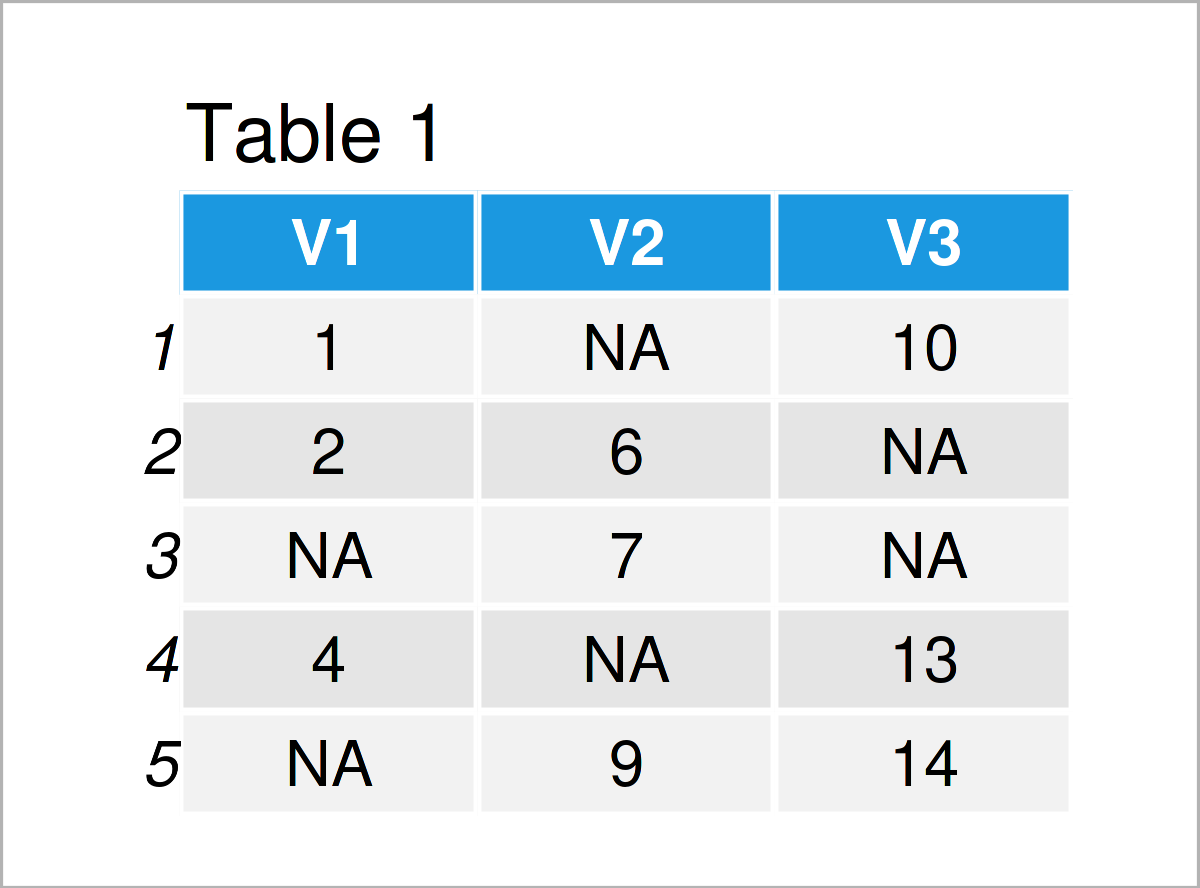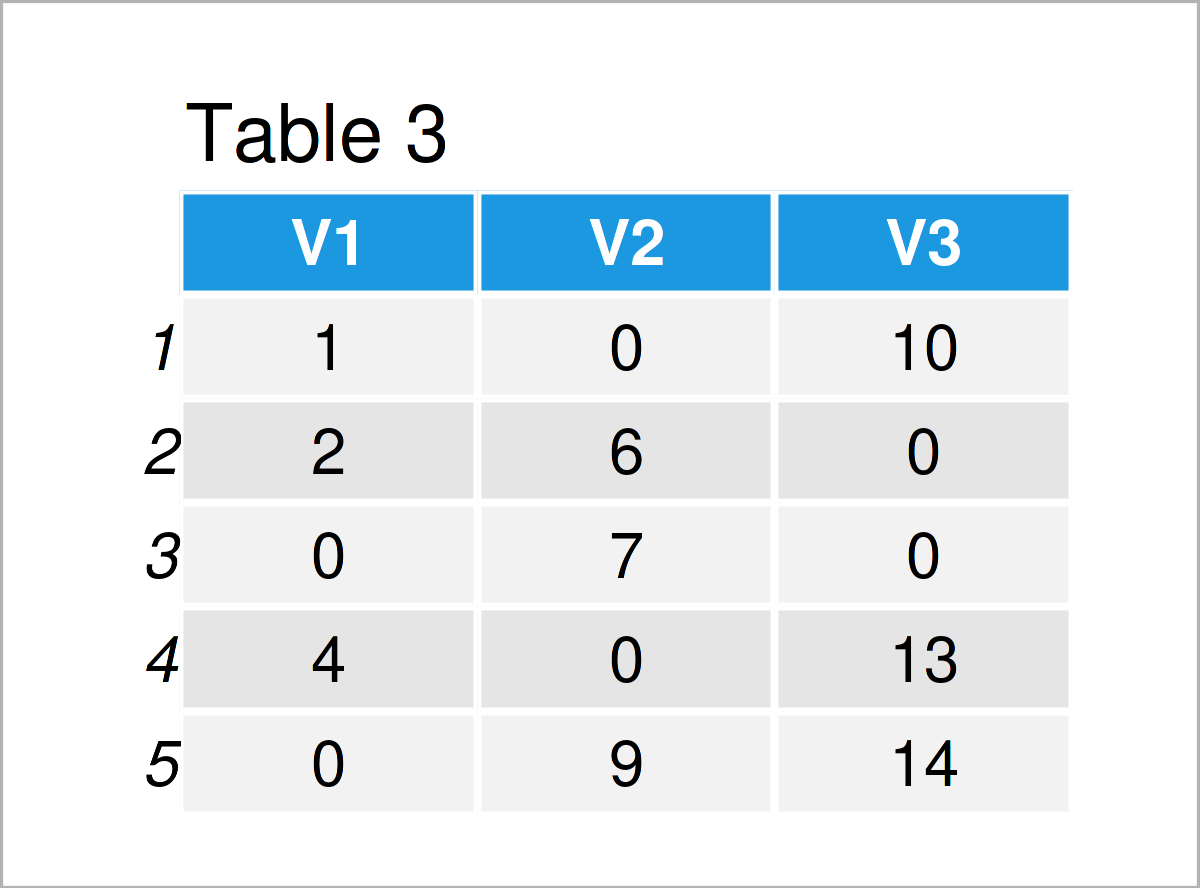# Replace NA in data.table by 0 in R (2 Examples)

This tutorial explains how to fill in NA values by 0 in data.table objects in the R programming language.

Let’s get started.

## Example Data & Packages

For this tutorial, we have to install and load the data.table package:

```install.packages("data.table")                  # Install & load data.table
library("data.table")```

We create some example data in form of a data.table object:

```data1 <- data.table(V1 = c(1, 2, NA, 4, NA),    # Create data.table
V2 = c(NA, 6, 7, NA, 9),
V3 = c(10, NA, NA, 13, 14))
data1                                           # Print data.table```As you can see based on Table 1, our example data data1 is a data.table consisting of five rows and three columns. All values are numeric. Some values are set to NA.

In many situations we have data with not only numeric, but also other kinds of values like strings or logical values. We therefore create a second dataset data2.

```data2 <- data.table(V1 = c(1, 2, NA, 4, NA),    # Create data.table
V2 = c(NA, "b", "c", NA, "e"),
V3 = c(FALSE, NA, NA, TRUE, TRUE))
data2                                           # Print data.table```After running the previous R code, the data.table object data2 shown in Table 2 was created. Again, some values were set to NA.

## Example 1: data.table with only numeric entries

In Example 1, I’ll explain how to fill in the NA values of data1 by 0.

```setnafill(data1, fill = 0)                      # Replace all NAs by 0
data1                                           # Print the edited data.table```With the above code all NA values in data.table object data1 were filled by 0. But be careful: When the data.table in which you want to replace NAs contains non-numeric columns, the above function setnafill will not work. Example 2 shows how to fill NA values for that case.

## Example 2: data.table with entries of different types (numeric, string, logical)

In Example 2, I’ll demonstrate how to fill all NA values of a data.table object which may includes non-numeric columns, for example strings. data2 is an example of such a data type.

```data2[is.na(data2), ] <- 0                      # Replace all NAs by 0
data2                                           # Print edited data.table```Table 4 shows the resulting edited data.table data2. For all different column types the NA values were set to 0.

## Video & Further Resources

I have recently published a video on my YouTube channel, which explains the content of this page. You can find the video below:

Furthermore, you could read some other R programming tutorials on this homepage. You can find some articles on similar topics such as vectors, missing data, and merging below:

This page was created in collaboration with Anna-Lena Wölwer. Have a look at Anna-Lena’s author page to get further information about her academic background and the other articles she has written for Statistics Globe.

Subscribe to the Statistics Globe Newsletter